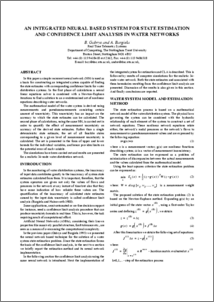# Integrated Neural Based System for State Estimation and Confidence Limit Analysis in Water Networks.

Gabrys, B. and Bargiela, A., 1997. Integrated Neural Based System for State Estimation and Confidence Limit Analysis in Water Networks. In: The 8th European Simulation Symposium, Ess 96. Society for Computer Simulation, 398-402.

Full text available as:Preview
PDF
Gabrys_Bargiela_1996_Int_SE_CL_Water_Systems.pdf - Accepted Version

54kB

## Abstract

In this paper a simple recurrent neural network (NN) is used as a basis for constructing an integrated system capable of finding the state estimates with corresponding confidence limits for water distribution systems. In the first phase of calculations a neural linear equations solver is combined with a Newton-Raphson iterations to find a solution to an overdetermined set of nonlinear equations describing water networks. The mathematical model of the water system is derived using measurements and pseudomeasurements consisting certain amount of uncertainty. This uncertainty has an impact on the accuracy to which the state estimates can be calculated. The second phase of calculations, using the same NN, is carried out in order to quantify the effect of measurement uncertainty on accuracy of the derived state estimates. Rather than a single deterministic state estimate, the set of all feasible states corresponding to a given level of measurement uncertainty is calculated. The set is presented in the form of upper and lower bounds for the individual variables, and hence provides limits on the potential error of each variable. The simulations have been carried out and results are presented for a realistic 34-node water distribution network.

Item Type: Book Section 978-1565550995 56 Faculty of Science & Technology 9644 Professor Bogdan Gabrys LEFT 11 Mar 2009 22:03 14 Mar 2022 13:21